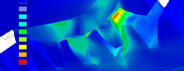﻿ ARA_ENG_UD041818 - Page 4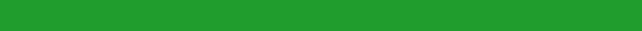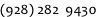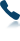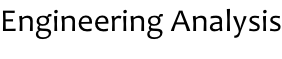Engineering analysis is the branch of engineering that uses computer simulations to understand the physics of engineered systems. Whether you are an entrepreneur wondering if your idea can work, or a large corporation that must sub-contract analysis, ARA Engineering, Inc. will provide the answers you need.

Engineering Analysis Includes:

Fluid flow (CFD): Computational fluid dynamics calculates transient and steady state fluid flows. This could be a gas, a liquid, or multiple phases including free surfaces.

Stress FEA (Structural): model the mechanical stresses in a system that is under load (either linear or non-linear and either due to a static load or a transient one)

Thermal FEA: model the flow of heat through a system (either steady state or transient and either linear or non-linear)

Creep Analysis of permanent deformation that accumulates while the load is applied

Normal Modes Analysis: determine the natural frequencies

Buckling analysis: predict the load that causes buckling

Transient Response: the response of a system to transient or time varying inputs. For example simulating a drop test or an earthquake.

Multiphysics Analysis: modeling a system that is in operation with many physical phenomena occurring. For example modeling simultaneous fluid flow and heat flow, or modeling fluid-solid interactions (FSI), or modeling thermally-induced transient stresses.

Kinematic and Dynamic Event Simulation: modeling a system that is in motion or otherwise operating as intended.Copyright ARA Engineering Inc.   2020        Created By LIVmoore

ARA Engineering can provide scientific analysis and analytical engineering to support product design or product improvement. This can reduce your time to deliver a product to the market and can reduce product development costs.

Our capabilities include:

• Computational Fluid Dynamics (CFD, Fluid-solid interactions, and multiphysics)
• Finite Element Analysis (FEA)
• Stress
• Thermal
• Dynamic
• Linear and non-linear
• Motion simulation and animation

• Optical Ray Trace Simulation: modeling the interaction of light and matter and simulating the performance of optical instrumentation.

• Mathematical Modeling: applying the math of physics to the performance of engineered systems

• We use multiple FEA and CFD solvers and choose the best one for your project